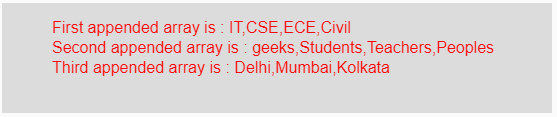GFG App
Open AppBrowser
Continue

# p5.js | append() function

The append() function in p5.js is used to add value at the end of a given array i.e, it increases the length of the original array by one.

Syntax:

`append(Array, Value)`

Parameters: This function accepts two parameters as mentioned above and described below:

• Array: It is the original input array to which a new value to be added.
• Value: It is the value which to be added at the end of the Array.

Return Value: It returns the new appended array.

Below program illustrates the append() function in p5.js:

Example: This example uses append() function to add any value at the end of the input array.

 `function` `setup() {  ` `  `  `    ``// Creating Canvas size ` `    ``createCanvas(550, 110);  ` `}  ` `  `  `function` `draw() {  ` `      `  `    ``// Set the background color  ` `    ``background(220);  ` `      `  `    ``// Initialize the array into variables ` `    ``let a = [``'IT'``, ``'CSE'``, ``'ECE'``]; ` `    ``let b = [``'geeks'``, ``'Students'``, ``'Teachers'``]; ` `    ``let c = [``'Delhi'``, ``'Mumbai'``]; ` `      `  `    ``// Initialize the value into variables ` `    ``let v = ``'Civil'``; ` `    ``let w = ``'Peoples'``; ` `    ``let x = ``'Kolkata'``; ` `    `  `    ``// Calling to append() function ` `    ``let p = append(a, v); ` `    ``let q = append(b, w); ` `    ``let r = append(c, x); ` `        `  `    ``// Set the size of text  ` `    ``textSize(16);  ` `      `  `    ``// Set the text color  ` `    ``fill(color(``'red'``));  ` `    `  `    ``// Getting appended array ` `    ``text(``"First appended array is : "` `+ p, 50, 30); ` `    ``text(``"Second appended array is : "` `+ q, 50, 50); ` `    ``text(``"Third appended array is : "` `+ r, 50, 70);           ` `}  `

Output:Reference: https://p5js.org/reference/#/p5/append

My Personal Notes arrow_drop_up